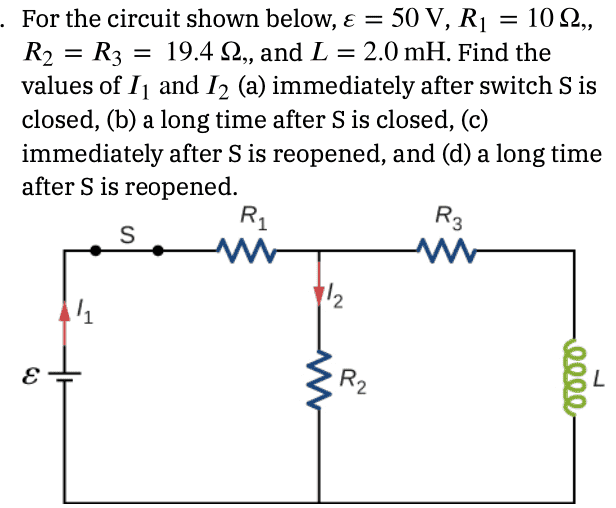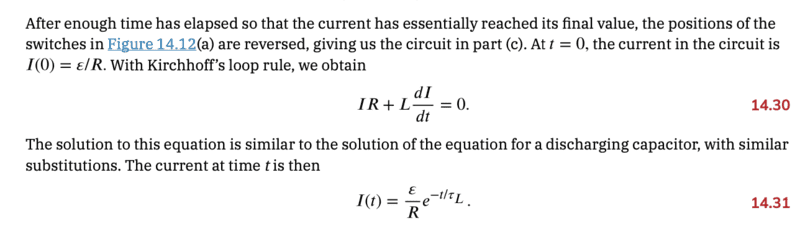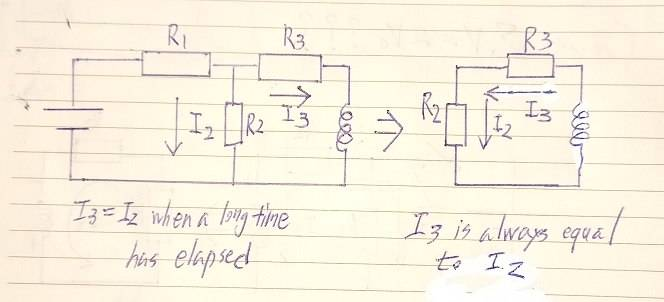# Current of RL circuit

• annamal

#### annamal

Homework Statement
For the circuit shown below, v = 50 V, R1 = 10 ohms, R2 = R3 = 19.4 ohms, and L = 2 mH. Find the values of I1 and I2 (c) immediately after S is reopened
Relevant Equations
##\frac{LdI_3}{dt} + I_2 R_3 + I_2 R_2 = 0##
##I_2 = \frac{V}{R_3 + R_2}e^{-t/\tau}##
##I_2 = \frac{V}{R_3 + R_2}e^{-t/\tau}##
##I_2 = \frac{50}{19.4(2)}e^{-t/\tau}## as t goes to 0, ##I_2 = \frac{50}{19.4(2)}## = 1.29 Amps which is not the answer.•Delta2

First of all, what is your reason for using that formula? Please motivate.

Also, how are ##I_3## and ##I_2## related? What is your definition of ##I_3##?

•bhobba and alan123hk
I think you do a similar mistake here as the one at the other thread (where you used ##\frac{\mathcal{E}}{R}## instead of ##\frac{\mathcal{E}}{2R}##)

For (c) that is immediately after S is reopened ##I_1## drops to zero and ##I_2## becomes equal to ##I_3##.
##I_3## can not change instantly because it is the current through the inductor, while we can make the assumption that the current through ##R_1## and ##R_2## can change instantly.

P.S As ##I_3## I refer to the current through the inductor (and ##R_3##) immediately before we commit action (c). That is the current through the inductor at the end of (b).

For (c) that is immediately after S is reopened I1 drops to zero and I2 becomes equal to I3.
OP has not yet defined ##I_3##. There is the matter of the sign if defined in the more canonical way. If so, ##I_3 \neq I_2##.

•bhobba
OP has not yet defined ##I_3##. There is the matter of the sign if defined in the more canonical way. If so, ##I_3 \neq I_2##.
This is interesting, can't wait for the OP to reply, so let's just say that ##I_3## is as I define it in my PS post #3.

This is interesting, can't wait for the OP to reply, so let's just say that ##I_3## is as I define it in my PS post #3.
That PS can be read as not necessarily specifying direction. I would say the canonical way would be to specify it as positive when the current goes from left to right through ##R_3##. Then clearly ##I_2 = - I_3## when the switch is off by Kirchhoff’s current law in the upper vertex (or lower).

Hmm sorry the truth is I am not expert in circuit theory so never heard of this "canonical way" of defining currents.

Hmm sorry the truth is I am not expert in circuit theory so never heard of this "canonical way" of defining currents.
I am just using canonical with its meaning of typical standard.

Hmm, this seems to me as a subtlety of no real consequence towards solving this problem but yes I think you are right after all, under proper definition of ##I_3## and ##I_2## it can be ##I_2=-I_3##.

I mean, not to give away too much, but one will have to deal with a minus sign somewhere.

Well yes ok, after reconsidering a bit , it is a subtlety of some consequence, the direction of ##I_2## is going to be reversed relative to what it is at the end of (b) and we have to express this in some way.

It is just meI am always thinking of currents as positive quantities in circuit theory. I ll have to review that.

It is just meI am always thinking of currents as positive quantities in circuit theory. I ll have to review that.
It's just you, and not for much longer, I'd guess. Yes, you need to get past that ASAP.

•Delta2
First of all, what is your reason for using that formula? Please motivate.

Also, how are ##I_3## and ##I_2## related? What is your definition of ##I_3##?
It says so in my book. ##I_3 = I_2##. Instead of I, we substitute for ##I_2##It says so in my book. ##I_3 = I_2##. Instead of I, we substitute for ##I_2##

View attachment 301753
This does not answer my question.

This does not answer my question.

What is your definition of I3?

That PS can be read as not necessarily specifying direction. I would say the canonical way would be to specify it as positive when the current goes from left to right through ##R_3##. Then clearly ##I_2 = - I_3## when the switch is off by Kirchhoff’s current law in the upper vertex (or lower).
I personally don't think there is a "canonical" way to assign polarities to voltages and currents in circuit analysis. However, every EE that does this has their own biases, like sources on the left and loads on the right. Currents flow left to right or clockwise in loops, voltages are more positive near the top, the passive sign convention, currents always flow into I/O ports, etc. The problem is that it doesn't take a very complex network before these rules fail, or, just don't seem simple.

One of the biggest error sources in circuit solutions is being confused about the polarity definitions and sign errors in the equations. I think the idea that there is a "right way" to define things actually makes this problem worse. It tempts people to think they don't have to worry about polarities or to make assumptions about the normal way it looks. A common problem is people trying to guess the answer to avoid negative values in their equation by defining things "the right way".

I think you are much better off to just understand up front the these assignments are COMPLETELY ARBITRARY, or could be. You always have to worry about this; you always have to write down your polarity definitions and refer back to them, you always have to TELL THE OTHER GUY what they are.

I think the idea that there is a "right way" to define things actually makes this problem worse.
I never claimed that there was a "right way", just that most people probably would assign ##I_3## to be the current going in the right direction (as in directional right, not as in being right right) through ##R_3##.
you always have to write down your polarity definitions and refer back to them, you always have to TELL THE OTHER GUY what they are.
Which is exactly why I asked OP for this clarification.

A common problem is people trying to guess the answer to avoid negative values in their equation by defining things "the right way", for example.
This is not unique to circuit theory. The same holds true in classical mechanics when people start drawing their forces. The good thing is that they will just come out negative if one assumes wrong regarding the sign.

The physical fact is that ##I_2## in (c), as defined in the image in the OP, will be negative regardless of how one defines ##I_3##.

•DaveE
What is your definition of I3?
I3 = I2

This is not unique to circuit theory. The same holds true in classical mechanics when people start drawing their forces. The good thing is that they will just come out negative if one assumes wrong regarding the sign.
Yes. I think it's a common point of confusion to students new to linear systems. We would all do well to stress to them that polarity definitions don't require you to know the answer in advance. I hear students getting stuck worrying about which to choose when it doesn't matter. If you want your arrows to point the right way, redraw them after you've solved it. I think students get mixed messages when the symbols are used in other contexts to show the actual direction of things like electrons, fields, etc. But in analysis, they are just a way to generate a consistent set of equations, nothing more.

What is your definition of ##I_3##?
I3 = I2
I'm quite sure, that really is not the sort of definition @Orodruin is looking for.

Assuming that I3 is the current flowing through resistor R3, in what direction is it assumed to be flowing?

What is your definition of I3?
I3 = I2
There seems to be a bit problem with this definition. Why define a ##I_3## if it is always equal to ##I_2##?
Why not just use the original ##I_2##, isn't it simpler and more convenient?

If ##I_3## is not always equal to ##I_2##, e.g. ##I_3=I_2## only after the switch ##S## is reopeoned, we cannot define that ##I_3 \cong I_2##, the position and direction of ##I_3## should be accurately marked on the circuit to avoid misunderstanding, although we might be able to infer the direction of ##I_3## from the relationship.

Edit : But you also didn't explicitly say that ##I_3## is equal to ##I_2## only after the switch is reopened.

Say you're running a business and communicating with customers. If it is not expressed clearly according to the established method, it will cause many problems.Last edited:
•DaveE, Delta2 and Steve4Physics
Here's an alternative approach.

The times of interest are the initial times (‘t=0’) and the steady-state times (‘t = ∞’). As a consequence, the questions are easily answered by understanding and applying three key facts about how an inductor behaves in a DC circuit like the one given:

1. For an ‘uncharged’ inductor, when the applied voltage changes suddenly, the inductor behaves (for an instant) as an infinite resistance (open circuit).

2. When the charging inductor reaches its steady state, it has zero voltage across it and a constant current (call it I₀) through it. The inductor acts as a zero resistance.

3. When the charged (steady-state) inductor discharges, the initial current through it is I₀ (as defined in point 2 above) and the final current through it is 0 (assuming no other sources are present).

If the above facts are applied, the questions can be easily answered without any differential equations. In fact this approach makes it obvious that the answers don’t even depend on the value of L!

Of course, the above approach won’t work for problems requiring currents at arbitrary specified times.

Can I also add to what @alan123hk said. The question does not ask for I₃. There is no reason to define it. The question asks only for I₁ and I₂ at key times. Using Kirchhoff’s 1st law, the current through R₃ and L (if required) is easily expressed in terms of I₁ and/or I₂ at the key times.

•alan123hk
Can I also add to what @alan123hk said. The question does not ask for I₃. There is no reason to define it. The question asks only for I₁ and I₂ at key times. Using Kirchhoff’s 1st law, the current through R₃ and L (if required) is easily expressed in terms of I₁ and/or I₂ at the key times.
Yes, it's not necessary to define ##I_3~##, but if the problem solver wants to define another variable ##I_3~##, that's fine too.
The problem now seems to be that OP mentioned a differential equation ##\frac{LdI_3}{dt} + I_2 R_3 + I_2 R_2 = 0~## involving ##I_3## in the first post, but he didn't explain clearly what it was, so it might confuse many people trying to follow his ideas to help.

•DaveE
Yes, it's not necessary to define ##I_3~##, but if the problem solver wants to define another variable ##I_3~##, that's fine too.
The problem now seems to be that OP mentioned a differential equation ##\frac{LdI_3}{dt} + I_2 R_3 + I_2 R_2 = 0~## involving ##I_3## in the first post, but he didn't explain clearly what it was, so it might confuse many people trying to follow his ideas to help.
I meant ##\frac{LdI_2}{dt} + I_2 R_3 + I_2 R_2 = 0## if that makes it clearer since I2 = I3. When I solve this differential equations, ##I_2 = \frac{V}{R_3 + R_2}e^{-t/\tau}##, but as t goes to 0, I2 = 1.29 amps which is not the answer.

•alan123hk
I meant LdI2dt+I2R3+I2R2=0 if that makes it clearer since I2 = I3. When I solve this differential equations, I2=VR3+R2e−t/τ, but as t goes to 0, I2 = 1.29 amps which is not the answer.
Ok, you mentioned in your first post that you were just focusing on finding an answer to question c ( immediately after S is reopened), which I seem to have missed.

Then we need to know what is the steady-state current of the inductor after the switch is closed for a long time, and this value is obviously not equal to ##\frac{V}{R_3 + R_2}=\frac{50}{19.4(2)}=1.29##•Delta2
I meant ##\frac{LdI_2}{dt} + I_2 R_3 + I_2 R_2 = 0## if that makes it clearer since I2 = I3. When I solve this differential equations, ##I_2 = \frac{V}{R_3 + R_2}e^{-t/\tau}##, but as t goes to 0, I2 = 1.29 amps which is not the answer.
You used wrong initial condition when solving the ODE. It is NOT $$I_2(0)=\frac{V}{R_3+R_2}$$ rather it is ##I_2(0)=##the current the inductor has at the end of (b) (that is immediately before (c) happens).

•alan123hk
Why define a ##I_3## if it is always equal to ##I_2##?
Not "always". The two currents are not equal after the switch is closed in part (a). They become equal when a "long time" has elapsed.

•SammyS
You used wrong initial condition when solving the ODE. It is NOT $$I_2(0)=\frac{V}{R_3+R_2}$$ rather it is ##I_2(0)=##the current the inductor has at the end of (b) (that is immediately before (c) happens).
I'd like to focus only on part c).
Why not? the L, R2, and R3 components are what makes the circuit after the circuit is immediately opened.

I'd like to focus only on part c).
Why not? the L, R2, and R3 components are what makes the circuit after the circuit is immediately opened.
The ODE you formed(at post #25) is correct. The general solution to that ODE is $$I(t)=Ce^{-t/\tau}$$ where $$\tau=\frac{L}{R_2+R_3}$$.

Now to determine the constant C you use as initial condition $$I(0)=\frac{V}{R_2+R_3}$$. I am going to throw the little ball to you now (direct translation from Greek idiomatic phrase e hehe). Please elaborate why you think that the initial current of inductor is that i.e ##\frac{V}{R_2+R_3}##, I mean, the voltage source V is detached from the loop when (c) happens, I really don't understand you here.

Not "always". The two currents are not equal after the switch is closed in part (a). They become equal when a "long time" has elapsed.
Yes, it does, because ##R_2=R_3##

Another interesting thing is that after removing the battery, according to the OP's statement ##I_3=I_2~##, ##I_3## needs to be defined as the opposite direction.Last edited:
•Delta2
Oops now I noticed that ##R_2=R_3##, however I think ##I_2(0)\neq\frac{V}{R_2+R_3}##, ##R_1## should feature in ##I_2(0)## too besides ##R_2,R_3##.

Oops now I noticed that ##R_2=R_3##, however I think ##I_2(0)\neq\frac{V}{R_2+R_3}##, ##R_1## should feature in ##I_2(0)## too besides ##R_2,R_3##.
But no current flows through R1...?

•Delta2
Good argument but still you are wrong. Hold on why I explain why.

What is the current through the inductor right before event (c)? Does ##R_1## feature in that? Can that current change instantly to ##\frac{V}{R_2+R_3}## right after event (c)?